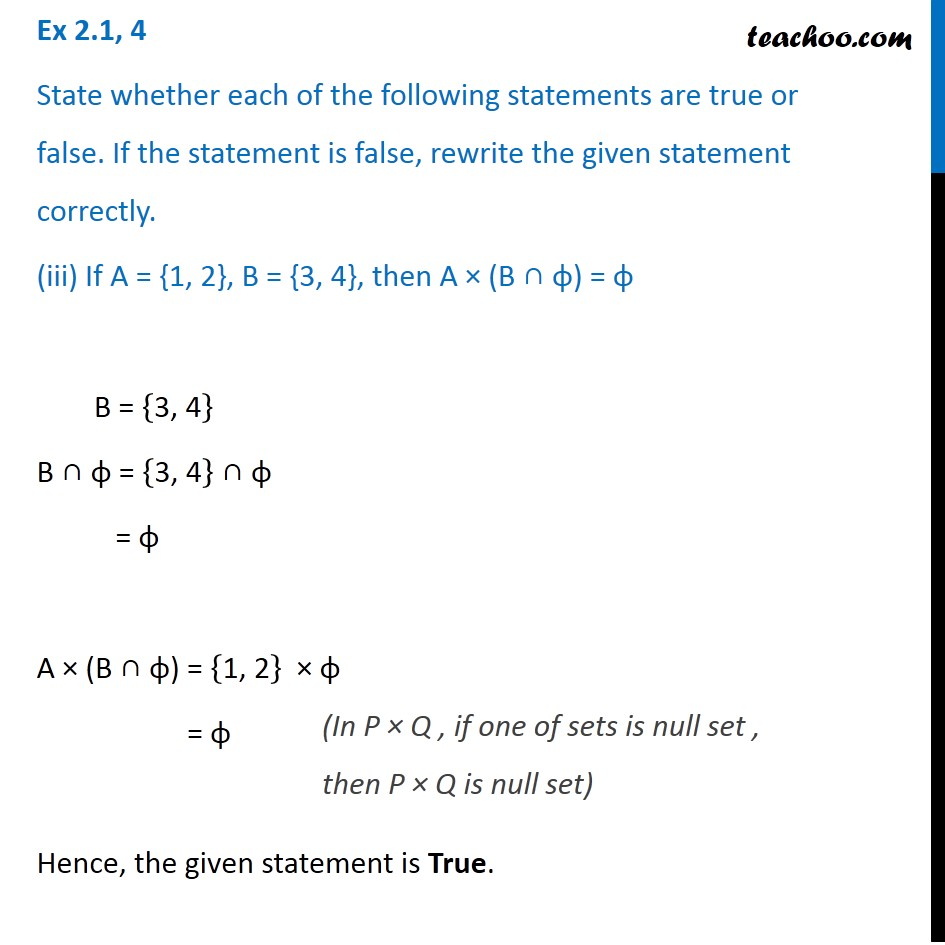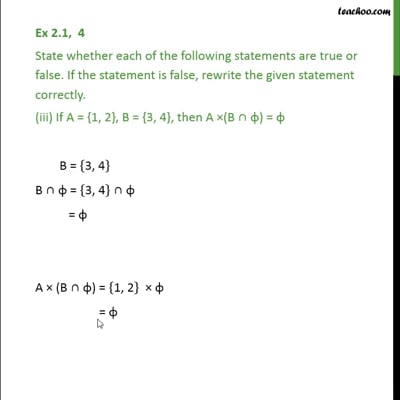Ex 2.1

Chapter 2 Class 11 Relations and Functions
Serial order wiseThis video is only available for Teachoo black users

Introducing your new favourite teacher - Teachoo Black, at only ₹83 per month

### Transcript

Ex 2.1, 4 State whether each of the following statements are true or false. If the statement is false, rewrite the given statement correctly. (iii) If A = {1, 2}, B = {3, 4}, then A × (B ∩ φ) = φ B = {"3, 4" } B ∩ φ = {"3, 4" } ∩ φ = φ A × (B ∩ φ) = {"1, 2" } × φ = φ Hence, the given statement is True. (In P × Q , if one of sets is null set , then P × Q is null set)